## General Chemistry

Galvanic cells are a type of electrochemical cell (also called voltaic cells and commonly known as batteries) where a spontaneous redox reaction generates an electric current. Another type of electrochemical cell is the electrolytic cell where, opposite to the galvanic cells, an electric current drives a nonspontaneous reaction. We will discuss the electrolytic cells in the next article, so, for now, the focus will be on the galvanic cells.

Now, before going into the mechanism of galvanic cells, let’s see what happens if we place a zinc plate in a Cu2+ solution such as CuSO4. Recall that Cu2+ solutions are known for a characteristic blue color, and interestingly, the presence of the zinc plate fades the color of the solution over time.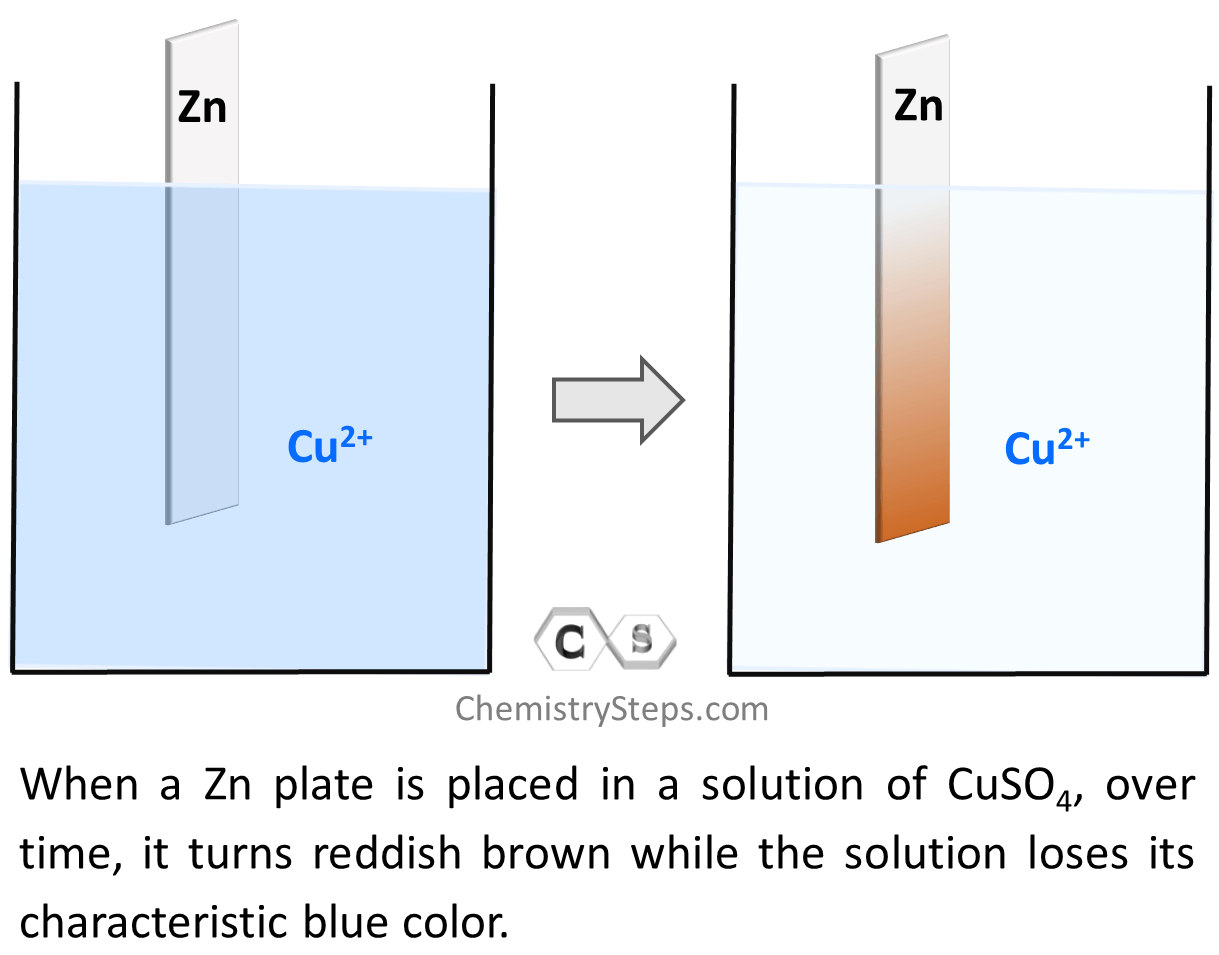So, what is the reason for the solution losing color?

Notice that on the right side, the zinc plate turns brownish red which is the color of Cu metal. This means there is Cu plating onto the Zn plate, and chemically speaking, we can say that Cu2+ ions are reduced to Cu. This, in turn, means that the Zn metal must be oxidized to Zn2+ because there cannot be a reduction of one specie whiteout another one being oxidized. The reducing electrons must come from somewhere, and the only possible source of them is the Zn metal. This is also confirmed by the increasing concentration of the Zn2+ ions which means the electrons are transferred from Zn to Cu2+ thus reducing them to Cu metal which deposits on the plate. The driving force of this electron transfer is the greater tendency of zinc to lose electrons compared to copper.The oxidation of Zn and reduction of Cu2+ species can be shown by the following two half-reactions, and by adding them we can get the overall redox reaction:

Zn(s) → Zn2+(aq) + 2e–

Cu2+(aq) + 2e → Cu(s)
_____________________________

Zn(s) + Cu2+(aq) → Zn2+(aq) + Cu(s)

And now, the next question: what does the reaction between Zn and Cu2+ have to do with galvanic cells? So, let’s find that out.

# Galvanic Cells

In the experiment described above, the Zn metal was in direct contact with Cu2+ ions and the electron transfer between them occurred right at their contact phase. Now, remember, electricity is a flow of electrons and therefore, if we could separate the Zn plate from the Cu2+ solution but find a way to support the electron flow between them, we’d generate electricity. For this, we make two separate solutions: one with a Zinc plate in a solution of its electrolyte, and the other with Cu in its electrolyte. And the important part, making the “remote” electron flow possible, is connecting the metals with a wire: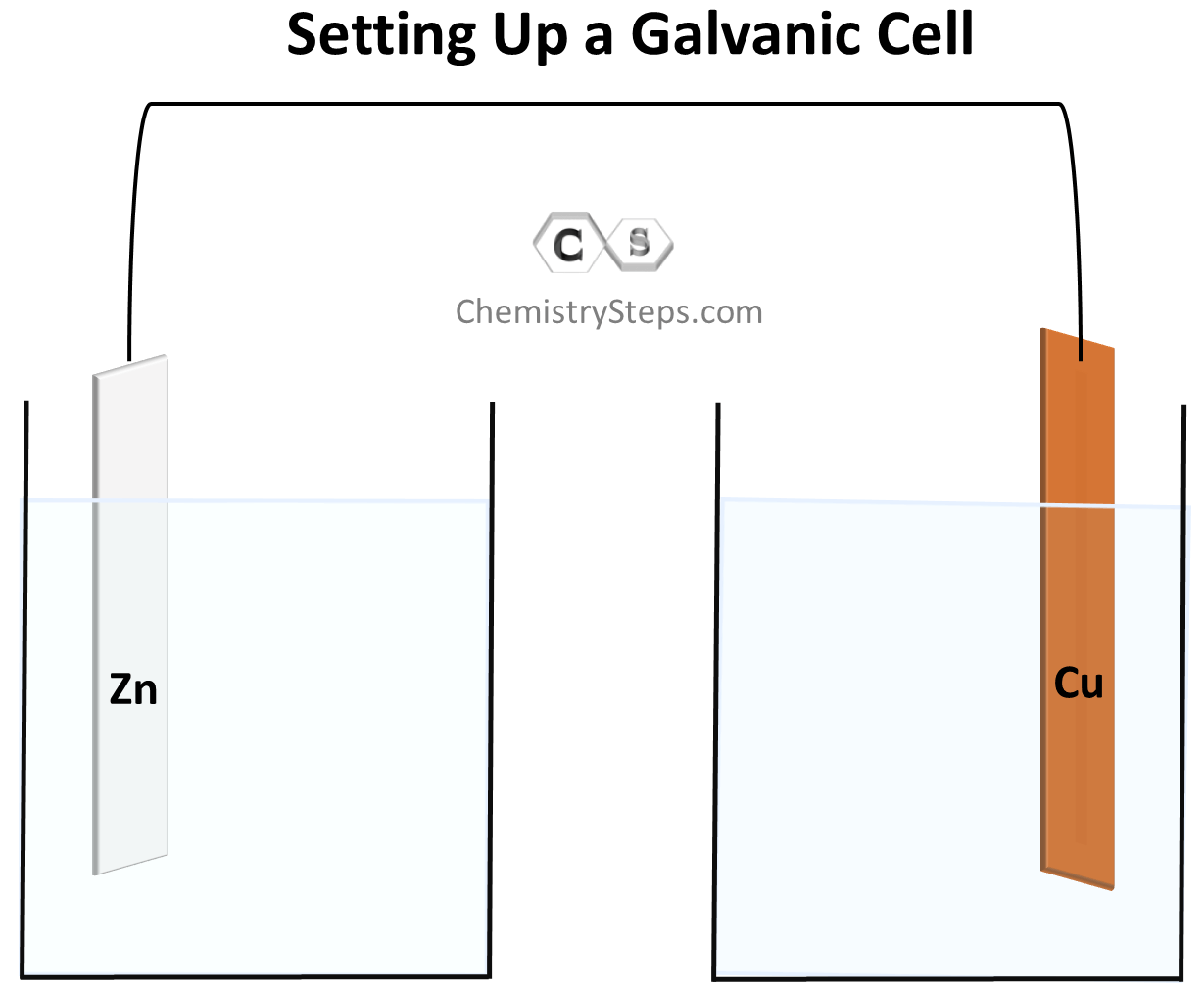As we have seen, Zn has a greater tendency to lose electrons and therefore, the electrons will start flowing to the Cu electrode. Because of the electron flow, Zn is oxidized to Zn2+, and Cu2+ is reduced to Cu according to the half-reactions we discussed earlier. The electrons forming as a result of the Zn to Zn2+ oxidation are accumulated on the metal and therefore, this electrode (anode) where the oxidation takes place is considered to be negatively charged even though, based on the direction of the electron flow, it may seem to be positively charged. The electrons then move to the cathode where the reduction of Cu2+ ions takes place.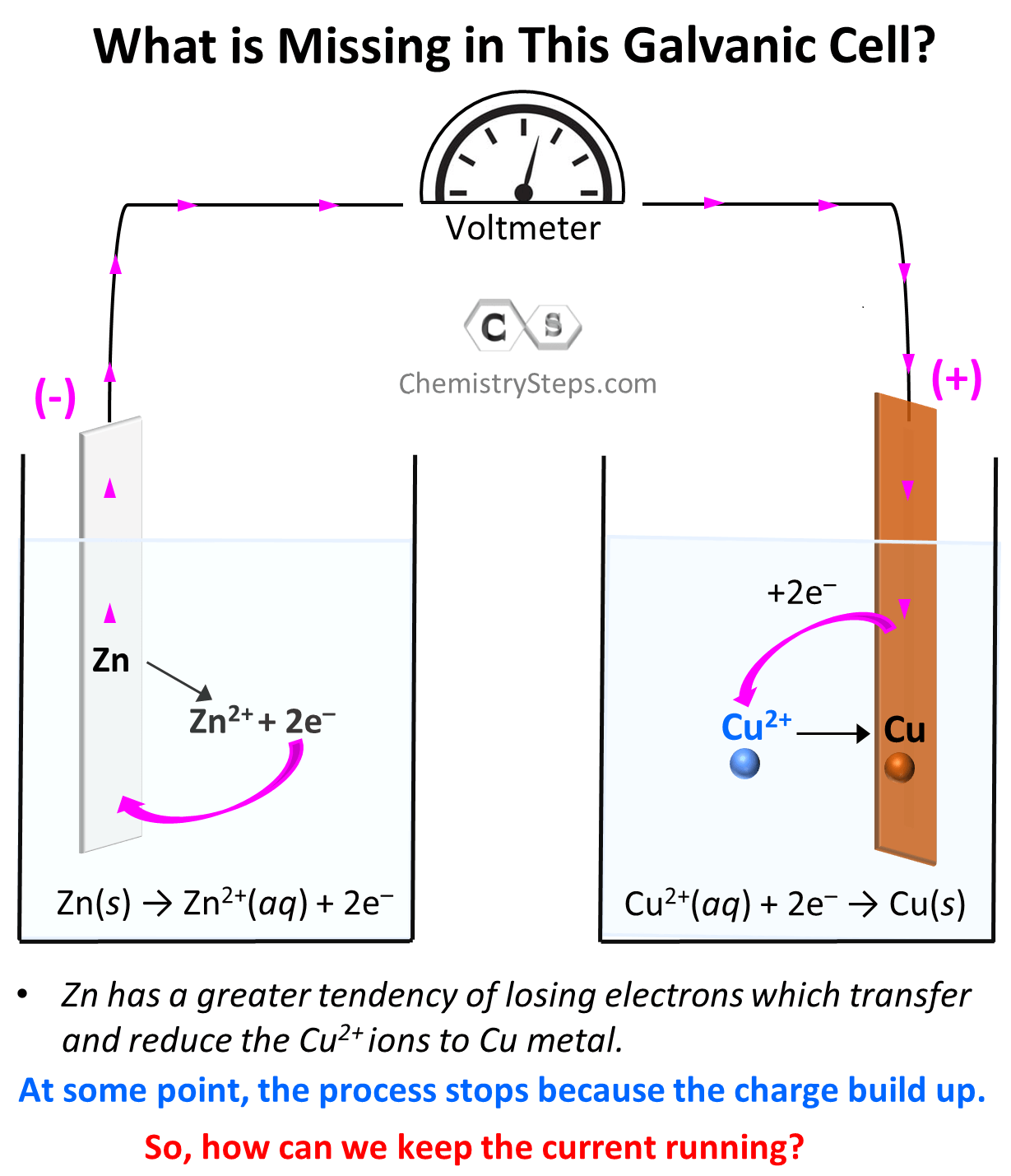The process continues till, at some point, the flow of electrons should stop because there cannot be an infinite accumulation of opposite charges on the two electrodes. Therefore, there must be a way of compensating the charge imbalance which is achieved by using a salt bridge. Which is a tube filled with a salt, ideally containing the anion of the electrolyte in both cells. As the current flows, the anions (SO42) move through a membrane to the solution containing the anode because it is being filled by Zn2+ cations, and the K+ ions move to the solution containing the cathode and balance the inflow of negatively charged electrons which drag the Cu2+ ions of the solutions. So, the salt bridge supports the current flow, and electricity is generated until there is no potential left between the two metals.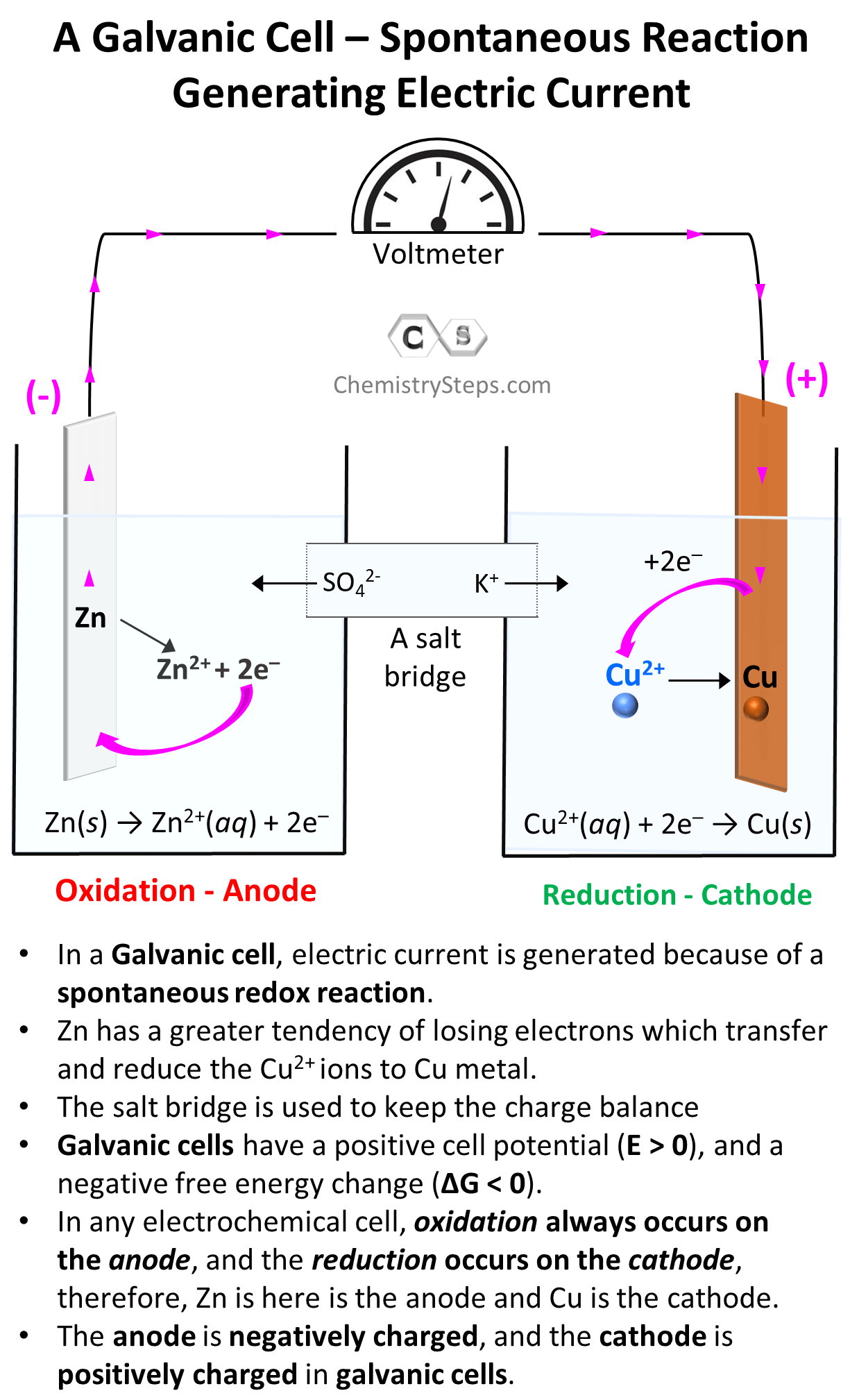In any electrochemical cell, oxidation always occurs on the anode, and the reduction occurs on the cathode, therefore, Zn is here is the anode and Cu is the cathode. In galvanic cells, the anode is considered to be negatively charged since it is accumulating the electrons before giving them to the cathode, while the cathode is positively charged as it gives those electrons to the cation in the solution.

Note: the charges are different in electrolytic cells!

# The Cell Potential

Each of the half-reactions is quantified with a characteristic cell potential (E) given in Volts. The cell potentials for the oxidation of Zn and reduction of Cu2+ are given below next to the corresponding half-reaction:

Zn(s) → Zn2+(aq) + 2e–   Eo = +0.76 V

Cu2+(aq) + 2e → Cu(s)   Eo = +0.34 V

The Eo notation indicates standard conditions which means the species in the solution (Zn2+ and Cu2+) are 1 molar, and the reaction is carried at 25 oC at 1 atm pressure.

By adding the two half-reactions, we can obtain the overall reaction and its cell potential

Zn(s) + Cu2+(aq) + 2e  → Zn2+(aq) + Cu(s)  + 2e

Zn(s) + Cu2+(aq) → Zn2+(aq) + Cu(s) Eo = +1.10 V

In the next article, we discuss calculating the cell potential in more detail.

# Electrochemical Cell Notation

The last part of today’s discussion will be cell notation or cell diagram which is compact notation of each species found in the two cells.

The cells are separated by a double line, while the species in each cell are divided by a single line which also indicates that they are in different phases.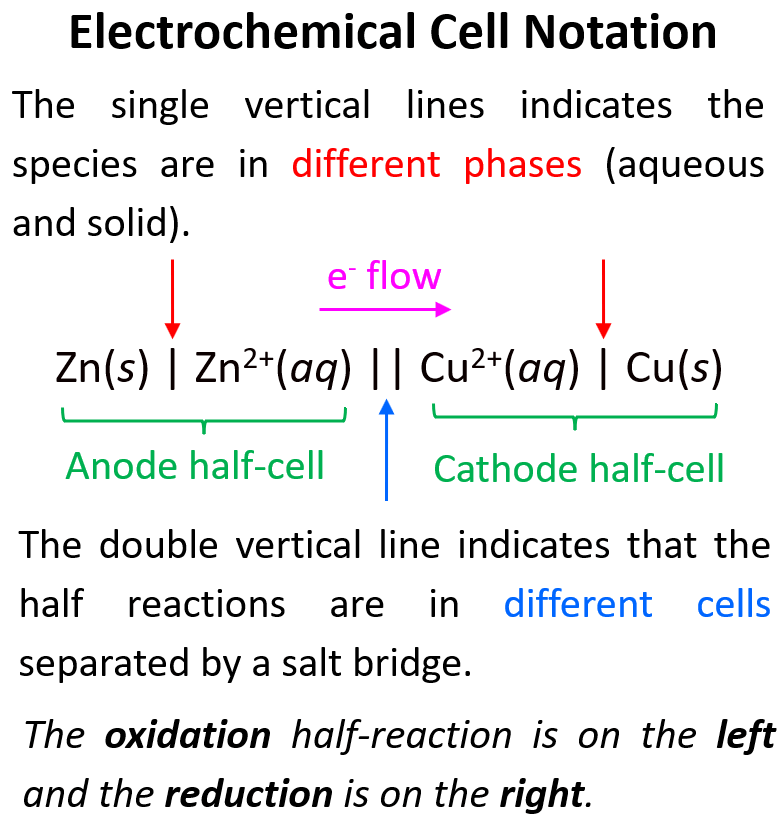Sometimes, the reactants and products of one or both of the half-reactions may be in the same phase. The reactants and products, in these reactions, are separated from each other with a comma. To support the current flow, an inert electrode, such as platinum (Pt) or graphite, is used and the anode and/or cathode is separated with a single vertical line in the line diagram.

One such example can be the oxidation of Fe to Fe2+ by potassium permanganate:

5 Fe(s) + 2 MnO4 (aq) + 16 H+(aq) → 5 Fe2+(aq) + 2 Mn2+(aq) + 8 H2O(l)

Anode – oxidation: Fe(s) → Fe2+(aq) + 2e

Cathode – reduction: 2 MnO4 (aq) + 8 H+(aq) + 5e→ 2 Mn2+(aq) + 4 H2O(l)

The electrons are transferred from the Fe anode to a Pt electrode merged in the solution of KMnO4:

Fe(s) | Fe2+(aq) || MnO4 (aq), H+, Mn2+(aq) | Pt(s)

To summarize what we learned about galvanic cells, remember that:

• In a Galvanic cell, electric current is generated because of a spontaneous redox reaction.
• Zn has a greater tendency of losing electrons which transfer and reduce the Cu2+ ions to Cu metal.
• The salt bridge is used to keep the charge balance
• Galvanic cells have a positive cell potential (E > 0), and a negative free energy change (ΔG < 0).
• In any electrochemical cell, oxidation always occurs on the anode, and the reduction occurs on the cathode, therefore, in our example, Zn is the anode and Cu is the cathode.
• The anode is negatively charged, and the cathode is positively charged in galvanic cells.